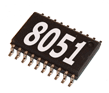# 8051 code find sum of first N natural numbersHello, guys!
Today we will see 8051 assembly program to find the sum of first N natural numbers. There are two ways to compute the sum of N natural numbers. We will go through one by one.
First method: It’s simple basic code. Let’s go through the algorithm.
Algorithm:

1. Start
2. Store the value(N) up to which sum has to be found in a register(Ra)
3. Initialize a register(Rb) to 1
4. Enter the loop
• Add Rb and Accumulator register(A) and store the value in A
• Increment Rb
• Decrement and check whether Ra is zero
• If Ra is zero exit the loop and move value stored in A to destination register(Rd)
• Else, jump back and repeat the steps in loop
5. Stop

here is an example code to find the sum of first 10 natural numbers.
Code:

Second method: Using formula, It’s simple and faster way to compute the sum using formula
Sum=n(n+1)/2
No need of algorithm. Example code is showed below
Code:
Important: When to use which algorithm?
Use the first method when your N value is small so that machine cycles reduces. And use the second method when N value is large so that you can get the result at a faster rate.
I have discussed both the methods in order to give you an idea about optimization. We will discuss more about optimization in future posts.]

### Edit:

Apologies if I was not clear in explanation part.
I have discussed the possibilities of achieving the sum of first N natural numbers. In the first method, your program size is reduced but you will have to compromise on execution time for large numbers (say numbers with 8bit size and above).  Of course, the second method is simple to understand and remember. Use the second method to save time (Remember this method takes same execution time irrespective of the value of N).